# python中groupby函数详解（非常容易懂）

## 一、groupby 能做什么？

python中groupby函数主要的作用是进行数据的分组以及分组后地组内运算！

df[](指输出数据的结果属性名称).groupby([df[属性],df[属性])(指分类的属性，数据的限定定语，可以有多个).mean()(对于数据的计算方式——函数名称)

print(df["评分"].groupby([df["地区"],df["类型"]]).mean())
#上面语句的功能是输出表格所有数据中不同地区不同类型的评分数据平均值

## 二、单类分组

A.groupby("性别")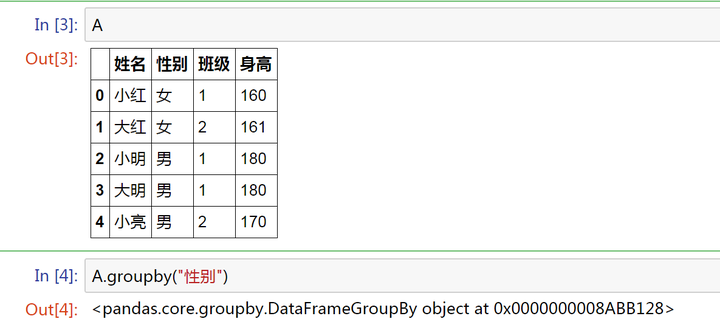## describe()

A.groupby("性别").describe().unstack()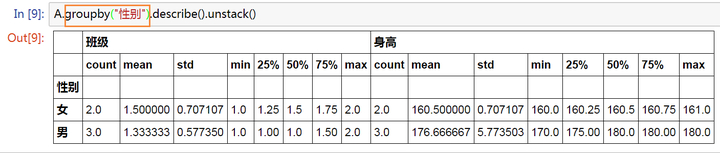* 只有数字类型的列数据才会计算统计

* 示例里面数字类型的数据有两列 【班级】和【身高】

A.groupby("性别")["身高"].describe().unstack()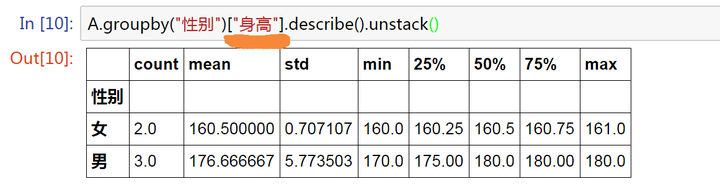## 三、多类分组

A.groupby( ["班级","性别"])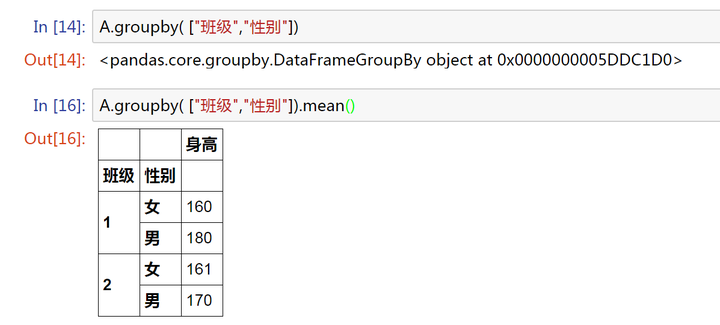## mean()

DataFrame的很多函数可以直接运用到Groupby对象上。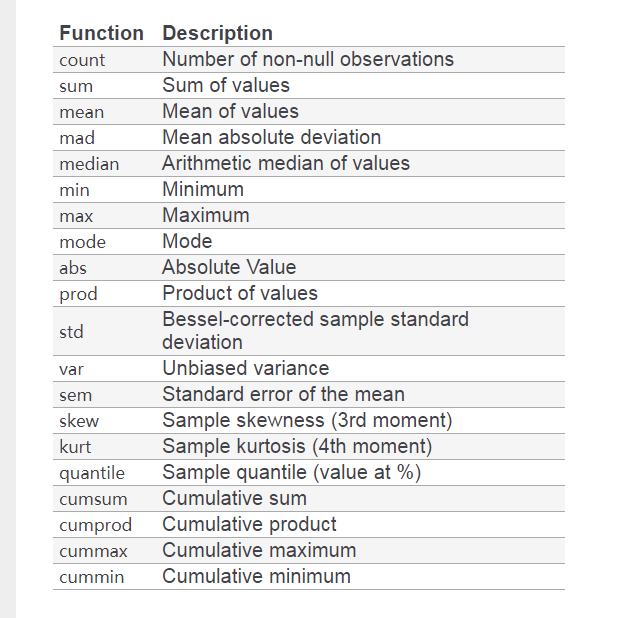A.groupby( ["班级","性别"]).agg([np.sum, np.mean, np.std]) # 一次计算了三个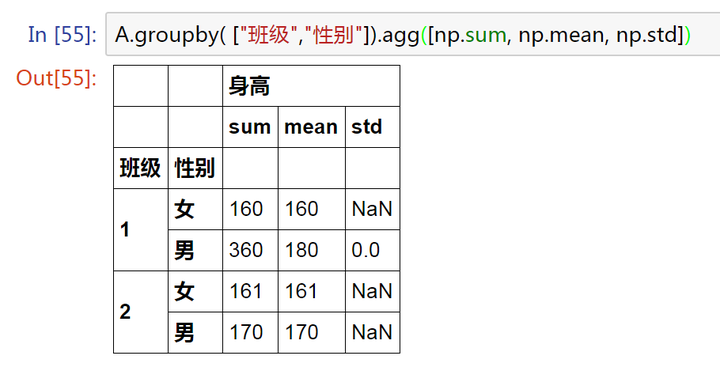## 四、时间分组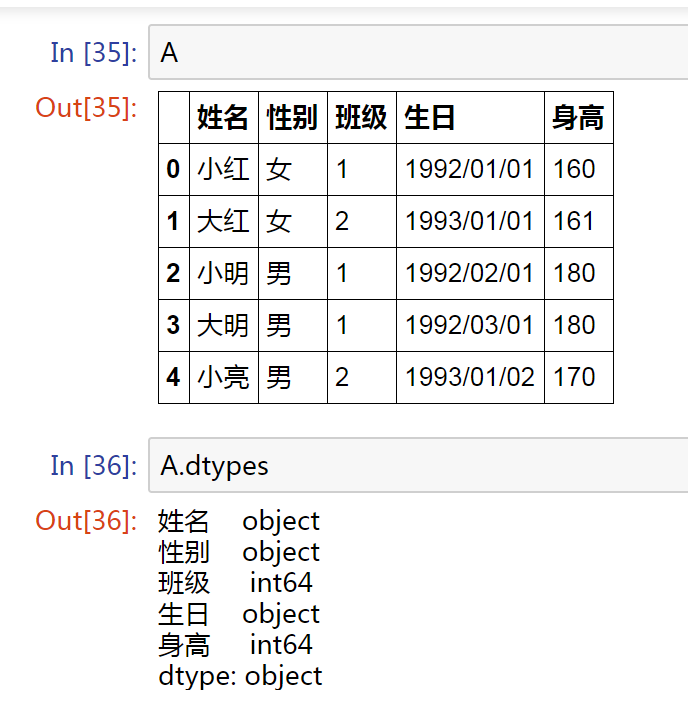1、按照【生日】的【年份】进行分组，看看有多少人是同龄？

A["生日"] = pd.to_datetime(A["生日"],format ="%Y/%m/%d")  # 转化为时间格式
A.groupby(A["生日"].apply(lambda x:x.year)).count() # 按照【生日】的【年份】分组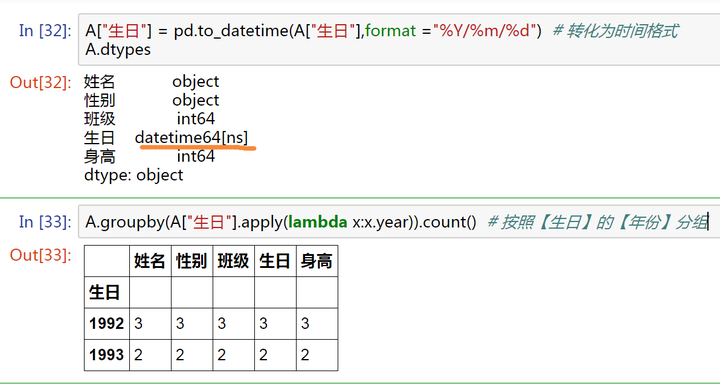2、同一年作为一个小组，小组内生日靠前的那一位作为小队长：

A.sort_values("生日", inplace=True)  # 按时间排序
A.groupby(A["生日"].apply(lambda x:x.year),as_index=False).first()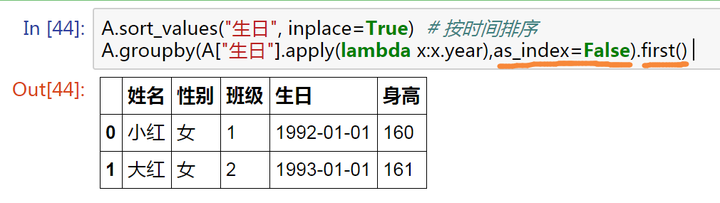## Tail(n=1)

3、想要找到哪个月只有一个人过生日

A.groupby(A["生日"].apply(lambda x:x.month),as_index=False)  # 到这里是按月分组
A.groupby(A["生日"].apply(lambda x:x.month),as_index=False).filter(lambda x: len(x)==1)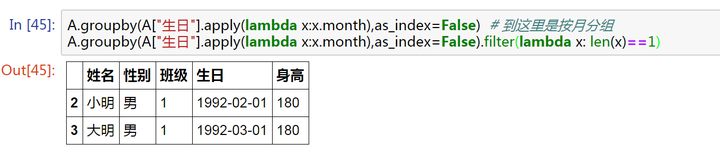## filter()

posted @ 2019-07-20 15:09  The-Chosen-One  阅读(164245)  评论(3编辑  收藏  举报